# Hubble Expansion and the Hypergeometrical Universe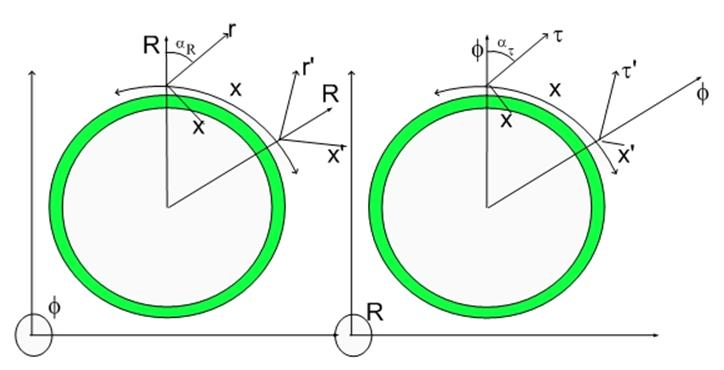Hubble Expansion and the Hypergeometrical Universe

Given that the Hypergeometrical Universe proposes a dynamic (lightspeed expanding hyperspherical Universe) topology for our Universe, it shouldn't be surprising that we have to revisit the interpretation of the Hubble constant and how one measures distance across the vast expanses.

Figure 1 displays the geometry for peering into the past. From the past blog, we established that the actual speed of light is sqr(2) times the observed speed of light.

I struggled a lot with this problem because it requires a Gedanken Experiment which I wasn't 100% sure about the outcome. The question is:
1. Can one make waves on a wave and see them propagating along the wave?
2. Can two pilots traveling in an open cockpit at the speed of sound hear each other speaking?
These seemingly simple questions (for a fluid dynamicist) bothered me a lot. If the two pilots could not hear each other that would imply that the speed of light is actually faster (sqr(2)* c) than the observed speed of light.

When I first derived the "solution" to this problem I simplified the problem just by considering a static hyperspherical reference frame. That introduced another constant into the problem (pi/3). The reason was the at any given Cosmological Angle, light travels in straight lines through the 4D spatial manifold, while we traveled along an arc. By the time light traveled all the distance to the 4D Center of the Universe, we would have traveled around the arc on an equilateral triangle inscribed inside a circle. I have to confess that this seems to be an artifact of how I tried to solve that problem. It gives a slightly older Universe than what we obtain when using the proper geometry:

The full treatment of the Pioneer Anomaly problem doesn't seem to require that constant.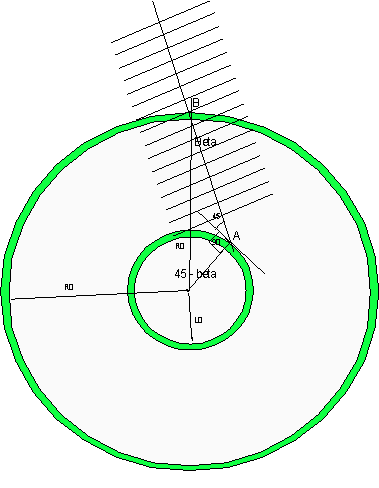Figure 1. Looking deep into the past.

This picture is quite telling. It shows the L0 radius of curvature of the time when the first stars lightened. At that time and at that radius of curvature, There was light… It is important to notice that light always travels at 45 degrees with the Fabric of Space – 45 degree with the tangential line at point A.

Under those conditions one can write the following equations for the relating L0, R0, Beta, LambdaObseved, CObserved – yes even the speed of light depends upon what are you looking at…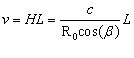Let's start with the speed of light. Normally we are observing things close, in our neighborhood… solar system, galaxy… Under those conditions, beta is 45 degrees and point A is almost right radially aligned with B. The two hyperspheres look like two hyperplanes and can be represented by the figure below: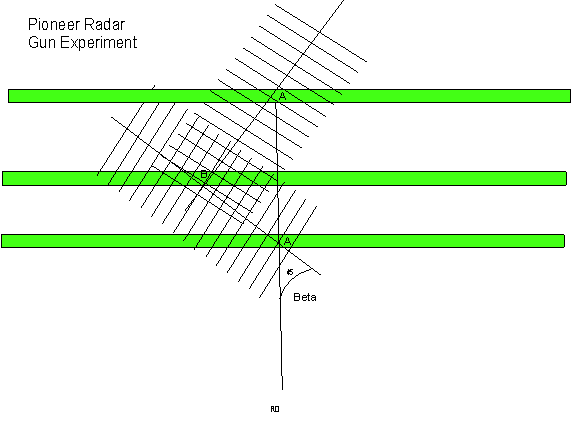Figure 2. Pioneer Radar Gun Experiment. Looking into a few minutes in the past.

This picture shows the Pioneer experiment. Light, microwaves leave Earth on point A and bounce back from the Pioneer spacecraft on point B. This all happens as the Hyperspherical Ligthspeed Expanding Universe expands at velocity Cobserved. The observed value for the speed of light is c, what we are used to think at the velocity of light. It just happens that the observed velocity depends upon the angle of observation. If you are observing the beginning of times, them the angle Beta will be very small, otherwise it will be around 45 degrees.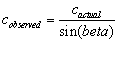where beta ranges from 45 degrees to zero degrees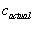is the actual speed of light which is faster than the speed of light we are used to… by a sqrt(2) factor.

Similarly, the wavelength of light depends upon what is the angle beta of observation.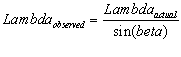This is expected, since the actual frequency of light shouldn't change. This also means that Hubble constant should depend upon how you calibrate it. Calibration is done using parallax and red shift or stellar candles and red shifts. These two calibrations are inconsistent since they look at different angles. One should use the near field calibration and then extrapolate it using the hypergeometrical argument.

Hubble equation relates distance with the receding velocity: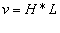The Shifted Frequency is related to Hubble constant by the following equation: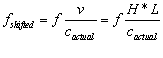Doppler effect equation.

The value of H is obtained when L is Ro. Under those considerations v becomes Cactual: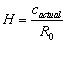In summary, here we presented a geometric model for the Doppler Shift, that is, the change in wavelength has only to do with the 4D angle of observation. This angle cannot be drawn within the 3D Hypersphere since when looking into the past, one is looking outside the hyperspherical hypersurface.

Numerically, the modeled Hubble coefficient is a match to the experimental Hubble coefficient.

H=2.0637E-18 s^-1

Cheers,

MP

PS - The most recent observational determination of the proportionality constant obtained in 2009 by using the Hubble Space Telescope (HST) yielded a value of H0 = 74.2 ± 3.6 (km/s)/Mpc. The results agree closely with an earlier measurement of H0 = 72 ± 8 km/s/Mpc obtained in 2001 also by the HST. In August 2006, a less-precise figure was obtained independently using data from NASA's Chandra X-ray Observatory: H0 = 77 (km/s)/Mpc or about 2.5×10−18 s−1 with an uncertainty of ± 15%. NASA summarizes existing data to indicate a constant of 70.8 ± 1.6 (km/s)/Mpc if space is assumed to be flat, or 70.8 ± 4.0 (km/s)/Mpc otherwise.

1 megaParsec = 3.08568025 × 1022 meters

1megaparsec/Km=3.08568025 × 1019

Thus

H=2.0637E-18 s^-1=2.0637E-18 s^-1* 3.08568025 E19 km/s/mpc=63.63 km/s/mpc

Where discrepancies are likely due to NASA averaging values from different epochs (beta angles).

Currently unrated

### New Comment

required

required (not published)

optional

required